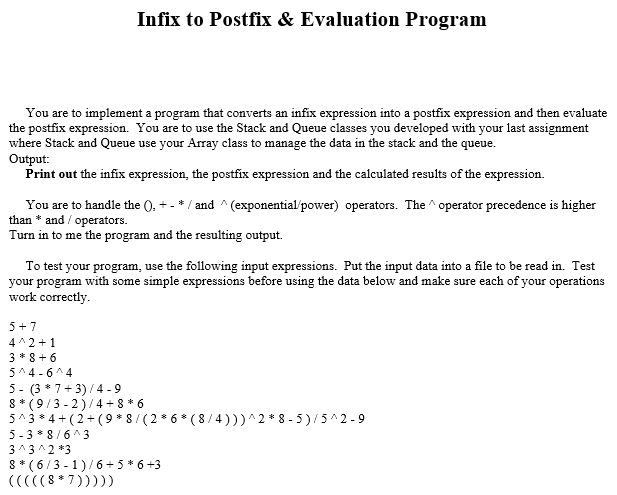Home / Answered Questions / Other / infix-to-postfix-evaluation-program-you-are-to-implement-a-program-that-converts-an-infix-expression-aw768

# (Solved): Infix To Postfix & Evaluation Program You Are To Implement A Program That Converts An Infix Expressi...

C++ please. Pay attention to we cannot use the standard template library.Infix to Postfix & Evaluation Program You are to implement a program that converts an infix expression into a postfix expression and then evaluate the postfix expression. You are to use the Stack and Queue classes you developed with your last assignment where Stack and Queue use your Array class to manage the data in the stack and the queue. Output: Print out the infix expression, the postfix expression and the calculated results of the expression. You are to handle the 0.+./and (exponential/power) operators. The operator precedence is higher than * and / operators. Turn in to me the program and the resulting output. To test your program, use the following input expressions. Put the input data into a file to be read in. Test your program with some simple expressions before using the data below and make sure each of your operations work correctly. 5+7 4^2+1 3* 8 + 6 5^4-614 5 - (3 * 7 + 3) /4-9 8* (9/3-2/4-8 * 6 5^3*4+(2+(9*8/(2*6*(8/4)))^2*8-5)/5^2-9 5-3 * 8/643 3^3^2*3 8*(6/3-1)/6-5*6+3 (((((8*7)))))

We have an Answer from Expert